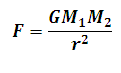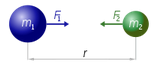# Newton's Law of Gravitation Calculator

This Calctown Calculator calculates the gravitational force of attraction between two bodies following newton's third la of motion.

kg
kg
m

#### Result

N